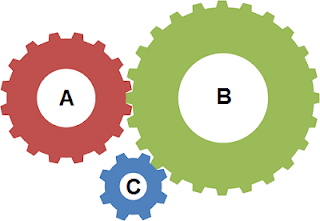# Ratio and Proportion 2-1

1. Divided Rs.600 among A,B and C so that Rs.40 more than $\displaystyle\frac{2}{5}$th of A's share. Rs.20 more than $\displaystyle\frac{2}{7}$ of B's share and Rs.10 more than $\displaystyle\frac{9}{{17}}$th of C's share may all be equal. What is A's share ?
a. Rs.280
b. Rs.150
c. Rs.170
d. Rs.200
Explanation:
$\displaystyle\frac{2}{5}A + 40 = \displaystyle\frac{2}{7}B + 20 = \displaystyle\frac{9}{{17}}C + 10 = x$
A = $\displaystyle\frac{5}{2}(x - 40),B = \displaystyle\frac{7}{2}(x - 20)$ and c = $\displaystyle\frac{{17}}{9}(x - 10)$
Given that $\displaystyle\frac{5}{2}(x - 40) + \displaystyle\frac{7}{2}(x - 20)$ + $\displaystyle\frac{{17}}{9}(x - 10) = 600$
$\displaystyle\frac{{45x - 1800 + 63x - 1260 + 34x - 340}}{{18}} = 600$
$45x - 1800 + 63x -1260 + 34x - 340$ $=10800$
$142x=14200$
$x = \displaystyle\frac{{14200}}{{142}} = 100$
Hence, A's share = $\displaystyle\frac{5}{2}(100 - 40) = Rs.150$

2. If 30 men working 7 hours a day can make 12 tables in 18 days, how many days will 45 women working 9 hours a day take to make 32 chairs? Given, 4 men can make 3 tables in the same time as 3 women can make 4 chairs.
a. 12 days
b. 14 days
c. 28 days
d. 21 days
Explanation:
We cannot directly apply chain rule here as work is different in both cases as well as on the first job men are working and on the second women are working.

How to think:
We first try to estimate the total work done by men in terms of tables, and total work done by women in terms of chairs also, we compare the works of men and women by using the last clue.  Take, women worked for d days to make 32 chairs.
30 men in 126 ( = 7 × 18) hours make 12 tables and 45 women in 9d hours make 32 chairs.
Let the assume 1 man capacity in 1 hour = m units, and 1 woman capacity in 1 hour = w units, One table = t units, one chair = c units.
Therefore,
$30m × 126 = 12t$ - - - (1)
$45w× 9d = 32c$- - - (2)
(1) ÷ (2),
$$\dfrac{{30m}}{{45w}} \times \dfrac{{126}}{{9d}} = \dfrac{{12t}}{{32c}}$$ - - - (3)

Given, 4 men can make 3 tables in the same time as 3 women can make 4 chairs.  Let us assume the time to complete these jobs = z hours
$4m×z = 3t$ - - - (4)
$3w×z = 4c$ - - - (5)
(4) ÷ (5), $$\dfrac{{4m}}{{3w}} = \dfrac{{3t}}{{4c}}$$
$$\Rightarrow \dfrac{m}{w} = \dfrac{{9t}}{{16c}}$$
Substituting the above value in equation (3),
$$\dfrac{{30}}{{45}} \times \dfrac{{9t}}{{16c}} \times \dfrac{{126}}{{9d}} = \dfrac{{12t}}{{32c}}$$
Interestingly, $$\dfrac{t}{c}$$ gets cancelled out.
$$d = \dfrac{{32}}{{12}} \times \dfrac{{30}}{{45}} \times \dfrac{9}{{16}} \times \dfrac{{126}}{9}$$ = 14

3. A wheel named "A" with 16 cogs is meshed with another wheel B with 24 cogs which in turn meshed with another wheel "C" with 8 cogs. If wheel 'A' makes 100 revolutions, how many revolutions will wheel "C" make?a. 70
b. 100
c. 200
d. 30
Explanation:
The number of revolutions made by the gears are inversely proportional to the number of cogs.
The ratio of $A : B = 16 : 24$.
So the number of revolutions are $24 : 16$
Similarly, the gears ratio of $B : C = 24 : 8$.
So the number of revolutions = $8 : 24 = 16 : 48$
Therefore, If gear A makes $24$ revolutions, gear $C$ makes $48$
For 1 revolution of A, Gear C makes 2
For 100 revolutions of A, gear C makes 200.

4. A box contains Red, Green and Blue balls.  The ratio of the number of red balls to green balls equals to the ratio of the number of Green balls to Blue balls.   If the total balls are 61 then find the number of green balls.
a. 7 : 3
b. 15 : 21
c. 10 : 21
d. 5 : 14
Explanation:
We generally attempt to solve this question like this. Given, $$R : G = G : B$$
So $${G^2} = RB$$
Also R + G + B = 61
How do we solve the above two equations?

How to think:
Instead of taking three variables, we try to solve this by taking only x, y.
Let us assume the ratio of Red to Green = $$x:y$$ = $$\left( {x:y} \right) \times x = {x^2}:xy$$
Given that Green to Blue = $$x:y$$ = $$\left( {x:y} \right) \times y = xy:{y^2}$$
Now, Red : Green : Blue = $${x^2}:xy:{y^2}$$
Given, $$\left( {{x^2}:xy:{y^2}} \right)k = 61$$
61 is a prime number.  So k = 1
Therefore, $${x^2} + xy + {y^2} = 61$$
So $${x^2} + 2xy + {y^2} = 61 + xy$$
$${\left( {x + y} \right)^2} = 61 + xy$$
$${\left( {4 + 5} \right)^2} = 61 + 20 = 81$$
Also, $x = 5$ and $y = 4$ also possible.
Therefore, number of Green balls = $xy = 20$.
Red and Blue are $16, 25$ or $25, 16$.  So we cannot uniquely determine their number.